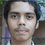# Trigonomentry Question

If sin A , cos A , tan A are in Geometric Progression . then $cot^{6} A - cot^{2}$

I wanted to know how? please Explain!Note by Vamsi Krishna Appili
6 years, 11 months ago

This discussion board is a place to discuss our Daily Challenges and the math and science related to those challenges. Explanations are more than just a solution — they should explain the steps and thinking strategies that you used to obtain the solution. Comments should further the discussion of math and science.

When posting on Brilliant:

• Use the emojis to react to an explanation, whether you're congratulating a job well done , or just really confused .
• Ask specific questions about the challenge or the steps in somebody's explanation. Well-posed questions can add a lot to the discussion, but posting "I don't understand!" doesn't help anyone.
• Try to contribute something new to the discussion, whether it is an extension, generalization or other idea related to the challenge.

MarkdownAppears as
*italics* or _italics_ italics
**bold** or __bold__ bold
- bulleted- list
• bulleted
• list
1. numbered2. list
1. numbered
2. list
Note: you must add a full line of space before and after lists for them to show up correctly
paragraph 1paragraph 2

paragraph 1

paragraph 2

[example link](https://brilliant.org)example link
> This is a quote
This is a quote
    # I indented these lines
# 4 spaces, and now they show
# up as a code block.

print "hello world"
# I indented these lines
# 4 spaces, and now they show
# up as a code block.

print "hello world"
MathAppears as
Remember to wrap math in $$ ... $$ or $ ... $ to ensure proper formatting.
2 \times 3 $2 \times 3$
2^{34} $2^{34}$
a_{i-1} $a_{i-1}$
\frac{2}{3} $\frac{2}{3}$
\sqrt{2} $\sqrt{2}$
\sum_{i=1}^3 $\sum_{i=1}^3$
\sin \theta $\sin \theta$
\boxed{123} $\boxed{123}$

Sort by:

$cos^{2}A=\frac{sin^{2}A}{cosA},\Rightarrow cot^{2}A=\frac{1}{cosA}.$As in question $cot^{6}A-cot^{2}A = \frac{1-cos^{2}A}{cos^{3}A} = \frac{sin^{2}A}{cos^{3}A} = 1.$

- 6 years, 11 months ago

right

- 6 years, 11 months ago

dear vamsi ,plz match the question and then post

- 6 years, 11 months ago

cos^2x=sin^2x!!!! Hence, cot^6x=cosec^2x!!! Therefore, by identity cosec^2x-cot^2x=1!!!!

- 6 years, 11 months ago

Calm down, that was understood without those exclamations also!

- 6 years, 11 months ago

what is this ^ ?

- 6 years, 11 months ago

^ means a number raised to some power like 2^2 means $\ 2^2$

- 6 years, 11 months ago